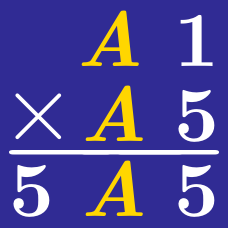Logic

# Algebraic Techniques - Cryptograms

$\begin{array}{ccccc} & & & & 1&3 \\ + & & & & \bigtriangledown &7\\ \hline & & & 1 & 0 &0 \end{array}$

Find the value of $\bigtriangledown$ satisfying the cryptogram above.

$\begin{array}{ccccc} & & & & \spadesuit&2 \\ & & & & \spadesuit&\spadesuit\\ + & & & & 1 &\spadesuit\\ \hline & & & & 7 &8 \end{array}$

Find the value that represents the symbol $\spadesuit$.

$\begin{array}{ccccc} & & & & 9 &P \\ - & & & & P & Q \\ \hline & & & & Q & 6 \end{array}$

Find the value of 2-digit integer $\overline{PQ}$.

$\begin{array}{ccccc} & & & & \color{#D61F06} A & B \\ + & & & & \color{#D61F06} C & D \\ \hline & & & & \color{#D61F06} E & F \end{array}$

The cryptogram above shows the sum of two 2-digit integers producing another 2-digit integer. In the leftmost column highlighted in red, which of the following cannot be true?

$\begin{array}{ccccc} & & & & 9 &5 \\ - & & & & 1 &\blacktriangle \\ \hline & & & & \blacktriangle & 8 \end{array}$

Find the value that represents the symbol $\blacktriangle$.

×